# States of Matter Notes Class 11 Chemistry Chapter 5 Download in P

7 States Of Matter, 5 States Of Matter, Different States Of Matter, Five States Of Matter, State Of Matter

Chapter 5 States of Matter

## States of Matter Notes Class 11 Chemistry Chapter 5 Download in Pdf

1.        Change in state : It is over all effect of Intermolecular forces, molecular Interactional energy & thermal energy:

2.       Measurable properties of gases  : P,V, T, n, Viscosity, specific heat are some measurable properties.

3.       Gas Laws : The quantitative relationship b/w any two of the variables (V, n, P,T) when other two are constant.

4.       Boyle’s Law : The pressure of fixed msss of gas varies inversely with the volume at constant T. P α 1/V(n,T const.) P1V1 = 2V 2

5.       Charle’s Law : At constant P, the volume of fixed amount of gas varies directly with its absolute temperature.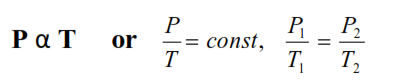6.       Gay lussac’s Law : At constant V, The pressure of fixedamount of gas varies directly with its absolute temperature.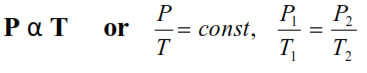7.       Ideal gas equation   : The relationship b/w P, V and T by Gas Laws  PV= nRT.

8.       Avogadro’s Law : At given T and P, the volume of gasvaries directly to the amount of gas .   V α n ( P, T constant)

9.       Dalton’s Law of partial persure :  The pressure enerted by a mixture of non reacting gases is equal to the sum of their partial pressure at constant (V,T)P (total ) = P1 + P2 + P3 + ………. (T, V, constant)

10.     Kinetic Molecular theory :

a.  Gases consist of large number of identical particles (atoms or molecules) that are so small that the actual volume of the molecules is negligible in comparison to the empty space between them.
b.  There is no force of attraction between the particles of a gas at ordinary temperature and pressure
c.  Particles of a gas are always in constant and random motion
d.  Pressure is exerted by the gas as a result of collision of the particles with the walls of the container
e.  Collisions of gas molecules are perfectly elastic
f.   At any particular time, different particles in the gas have different speeds and hence different kinetic energies
g.  Average kinetic energy of the gas molecules is directly proportional to the absolute temperature

11.     Real Gases : The gases which deviates from Ideal behavior at higher pressure and low temperature b/c of force of attraction b/w molecules increases .

12.     Compressibility factor (Z) : It determine extent of devation of real gases from Ideal gas behavior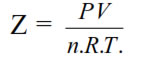for ideal gass formula gass Z=1, for Nonideal gas Z< 1, Z > 1

Vandor Walls equations:- The constants a and b have positive values and are characteristic of the individual gas. The van der Waals equation of state approaches the ideal gas law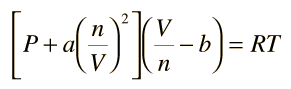14.     Critical Temperature : (Tc) The temperature above which a gas cannot be liquefied whatever high pressure may be

15.     Critical Pressure : The minimum pressure required to liquity a gas at its critical temperature.

16.     Critical Volume : The volume of 1 mole of gat at Tc, Pc.

17.     Super cooled liquids : The liquids which are cooled to a temperature below its freezing point without freezing .

18.     Elastic Collision : The collisions in which no loss of K.E. only there is transfer of energy.

19.     Vapour pressure : The equilibrium pressure by vapour of liquid in a container at given temperature (T)

20.     At  higher altitude : The b.p. of water decreases b/c the atmospheric pressure is less than one atmosphere.

21.     Surface Tension   (V) : It is force acting per unit length perpendicular to the line drawn on the surface : (Nm-1) :  It decreases with increases in T, it increases with increase in external pressure, b/c of it falling drops of liquid are spherical, liquid in capillary tube rises.

22.     Viscosity (η) : It is resistance offered to the flow of liquid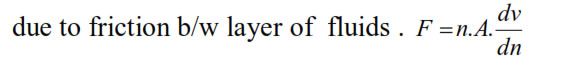23.     Effect of T & P on viscosity  : It decreases with increase inT, and increases with increase in P.

24.     Low  M.P.  &  B.P.  of  molecular  liquids  is  due  to  low magnitude of molecular interaction energy.

### NCERT Books Free Pdf Download for Class 5, 6, 7, 8, 9, 10 , 11, 12 Hindi and English Medium

 Mathematics Biology Psychology Chemistry English Economics Sociology Hindi Business Studies Geography Science Political Science Statistics Physics Accountancy